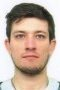# Site institutionnel du Laboratoire Ampère - CNRS UMR5005

## 15/06/2021 - Alexandre KIRCHER

published on , updated on

## Agenda

Alexandre Kircher defends his PhD on June 15, 2021 at 2:00 PM.
Place : visio conference only.

Design and Analysis of Resilient state estimators for Linear Discrete-time Systems.

Jury :
Rapporteurs :
ALESSANDRI Angelo, Professeur Associé, Università di Genova
ZASADZINSKI Michel, Professeur des Universités, Université de Lorraine (CRAN)

Autres membres :
ANDRIEU Vincent, Directeur de Recherche CNRS, Université Lyon 1 (LAGEPP)
LESECQ Suzanne, Directrice de Recherche, CEA Grenoble (List)
ZHANG Qinghua, Directeur de Recherche, INRIA Rennes

BAKO Laurent, Maître de conférences (HdR), Ecole Centrale de Lyon (Ampère)
BLANCO Eric, Maître de Conférences, Ecole Centrale de Lyon (Ampère)
BENALLOUCH Mohamed, Enseignant chercheur, ECAM LyonAbstract :
Given the role of state space representations in engineering methods linked with the control of systems, it is important to know the state of a system. However, there are systems for which the state is not directly accessible through measurement. The state estimation problem then consists in reconstructing the state of the system through the model of the system and accessible system measurement without knowing the exact values of the disturbances which can be present in the system.
In this PhD work, we aim at designing and analysing state estimators able to reconstruct the state of a system despite the presence of impulsive noises. This type of noise is characterised by having most of its entries equal to zero, but its nonzero entries can take arbitrarily large values. To do so, we will assess if the estimators verify the resilience property, which characterises an estimator whose performances are insensitive to a given class of disturbances. We will also investigate the approximate resilience property, a weaker version of resilience characterising when a given class of disturbances, potentially unbounded, have a bounded impact on the performances of the estimator.
Every estimator considered in this manuscript will be defined through the optimal estimation framework. An optimal estimator will be defined through the minimisation of a given cost function. The role of this cost function is to weight every hypothetical trajectory that the system state can potentially take. The estimator then returns the best state trajectories with respect to this cost function.

The choice of the cost functions is the first problem addressed in this manuscript. After reviewing the state of the art, we focus our attention on two interesting types of optimal state estimators. The estimators originating from the compressed sensing methods, which use norms in their cost function, form the first type. The second type refers to estimators deriving from the Maximum Correntropy Criterion (MCC) framework. These estimators are defined through cost functions presenting saturation due to the presence of exponential terms with negative arguments in their expression.
In order for the analysis to cover many estimators, the elements of these classes were defined through their properties, and not through a specific closed-form algebraic expression.

These classes were then analysed. The main theoretical results obtained in this PhD work are upper bounds on the norm of the estimation error, i.e. the difference between the real trajectory and the estimated trajectory. The existence of these bounds is guaranteed through sufficient conditions linked to the observability of the system, to properties verified by the cost function and to the number of nonzero entries in the impulsive noises. Moreover, these bounds have two main advantages. First of all, they do not depend on the extreme values taken by the impulsive noises. Secondly, they are explicitly expressed with the help of the different parameters of the estimators. This allows us to discuss how these parameters impact the quality of the estimation.

Finally, we will deal with the implementation of the estimators defined in this thesis. In the case where the estimator is defined through a convex cost function, it can be directly implemented through an algorithm using convex optimisation methods. We also derived several algorithms in order to approximately implement estimators defined through non convex cost function with exponential losses. In addition, we considered the recursive implementation of our estimators and derived Kalman-like recursive algorithms by applying the Forward Dynamic Programming (FDP) framework.
The performances of all the algorithms defined were assessed in simulation. In particular, we displayed four typical cases of applications. These cases, linked to actual engineering problems, aim at showcasing the versatility of the frameworks we developed.

Keywords :
Resilient State Estimation, Secured State Estimation, Optimal estimation, Recursive estimator, Forward Dynamic Programming, Impulsive noise, Sparse noise, Cyber-Physical Systems, Maximum Correntropy Criterion

View online : Texte complet